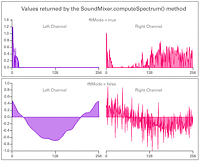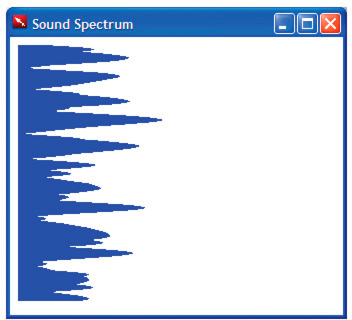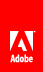# Accessing raw sound data

The SoundMixer.computeSpectrum() method lets an application read the raw sound data for the waveform that is currently being played. If more than one SoundChannel object is currently playing, the SoundMixer.computeSpectrum() method shows the combined sound data of every SoundChannel object mixed together.

## How sound data is returned

The sound data is returned as a ByteArray object containing 512 four-byte sets of data, each of which represents a floating point value between -1 and 1. These values represent the amplitude of the points in the sound waveform being played. The values are delivered in two groups of 256, the first group for the left stereo channel and the second group for the right stereo channel.

The SoundMixer.computeSpectrum() method returns frequency spectrum data rather than waveform data if the FFTMode parameter is set to true . The frequency spectrum shows amplitude arranged by sound frequency, from lowest frequency to highest. A Fast Fourier Transform (FFT) is used to convert the waveform data into frequency spectrum data. The resulting frequency spectrum values range from 0 to roughly 1.414 (the square root of 2).

The following diagram compares the data returned from the computeSpectrum() method when the FFTMode parameter is set to true and when it is set to false . The sound used for this diagram contains a loud bass sound in the left channel and a drum hit sound in the right channel.

View full size graphicThe computeSpectrum() method can also return data that has been resampled at a lower bit rate. Generally, this results in smoother waveform data or frequency data at the expense of detail. The stretchFactor parameter controls the rate at which the computeSpectrum() method data is sampled. When the stretchFactor parameter is set to 0, the default, the sound data is sampled at a rate of 44.1 kHz. The rate is halved at each successive value of the stretchFactor parameter. So a value of 1 specifies a rate of 22.05 kHz, a value of 2 specifies a rate of 11.025 kHz, and so on. The computeSpectrum() method still returns 256 floating point values per stereo channel when a higher stretchFactor value is used.

## Building a simple sound visualizer

The following example uses the SoundMixer.computeSpectrum() method to show a chart of the sound waveform that animates periodically:```<html>
<title>Sound Spectrum</title>
<script src="AIRAliases.js" />
<script>
const PLOT_WIDTH = 600;
const CHANNEL_LENGTH = 256;

var snd = new air.Sound();
var req = new air.URLRequest("test.mp3");
var bytes = new air.ByteArray();
var divStyles = new Array;

/**
* Initializes the application. It draws 256 DIV elements to the document body,
* and sets up a divStyles array that contains references to the style objects of
* each DIV element. It then calls the playSound() function.
*/
function init()
{
var div;
for (i = 0; i < CHANNEL_LENGTH; i++)
{
div = document.createElement("div");
div.style.height = "1px";
div.style.width = "0px";
div.style.backgroundColor = "blue";
document.body.appendChild(div);
divStyles[i] = div.style;
}
playSound();
}
/**
* Plays a sound, and calls setInterval() to call the setMeter() function
* periodically, to display the sound spectrum data.
*/
function playSound()
{
if (snd.url != null)
{
snd.close();
}
var channel = snd.play();
timer = setInterval(setMeter, 100);
}

/**
* Computes the width of each of the 256 colored DIV tags in the document,
* based on data returned by the call to SoundMixer.computeSpectrum(). The
* first 256 floating point numbers in the byte array represent the data from
* the left channel, and then next 256 floating point numbers represent the
* data from the right channel.
*/
function setMeter()
{
air.SoundMixer.computeSpectrum(bytes, false, 0);
var n;
for (var i = 0; i < CHANNEL_LENGTH; i++)
{
bytes.position = i * 4;
bytes.position = 256*4 + i * 4;
divStyles[i].width = n * PLOT_WIDTH;
}
}
/**
* When the sound is done playing, remove the intermediate process
* started by setInterval().
*/
function onPlaybackComplete(event)
{
clearInterval(interval);
}
</script>